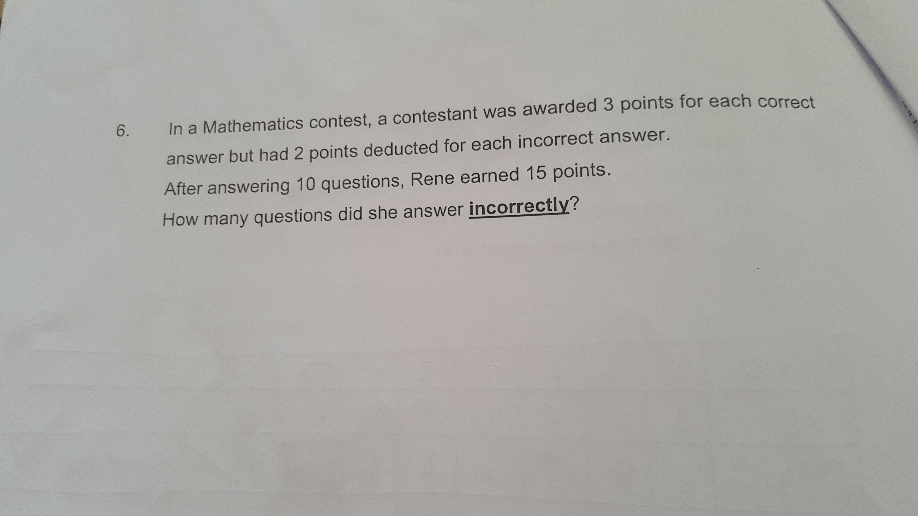# QuestionAppreciate you guys help😉.

2 Answers

# Answer

10 x 3 = 30 (assuming if she answered all 10 questions correctly)

30 – 15 = 15 (difference)

3 + 2 = 5 (points that she would have lesser for each question she answered incorrectly)

15/5 = 3

Ans : 3 questions.

To check :

7 (correct) x 3 = 21

3 (incorrect) x 2 = – 6

21 – 6 = 15 marks earned.

0 Replies 0 Likes